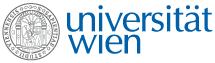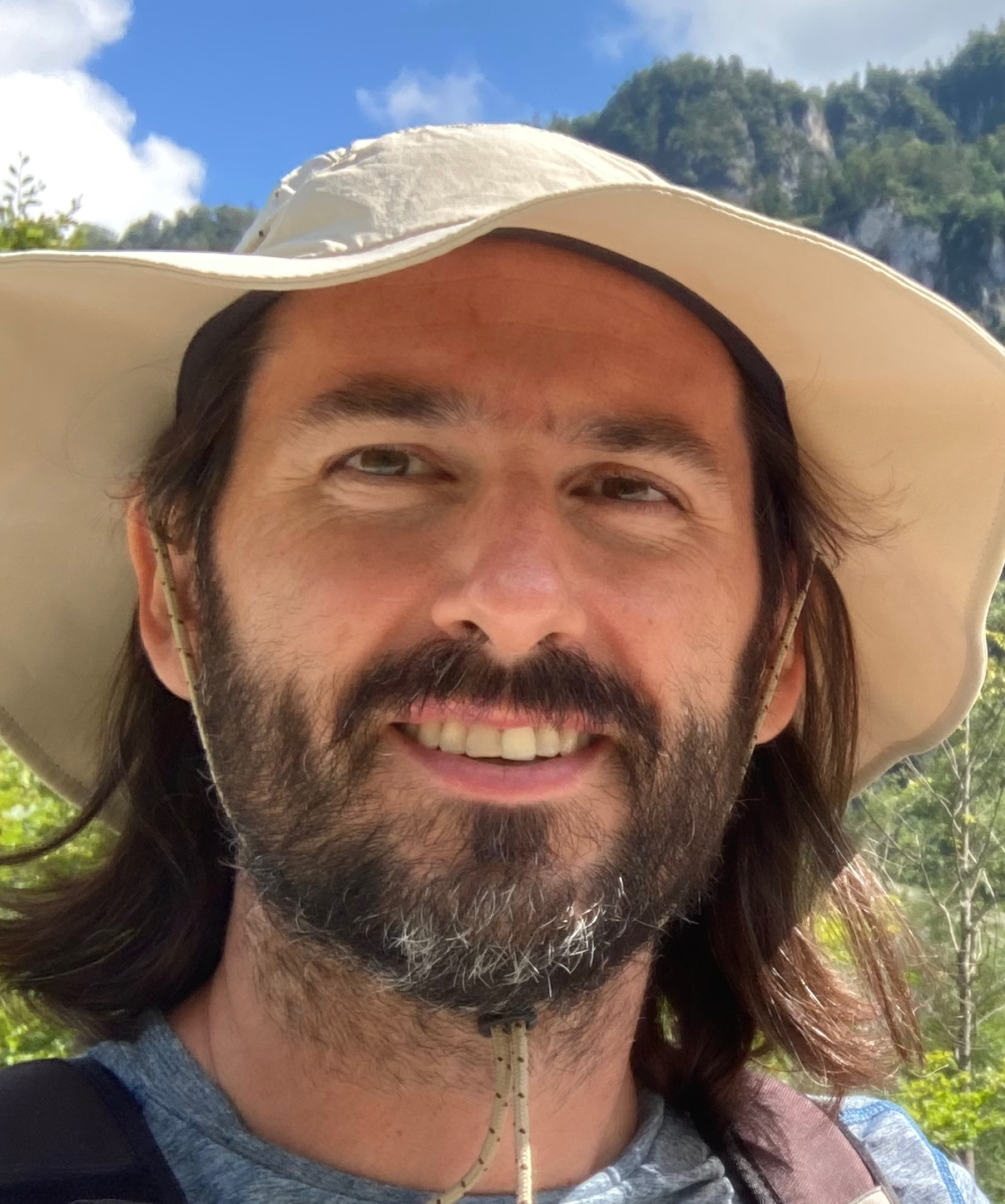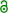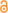# Christopher H. CashenChristopher H. Cashen, Privatdoz. PhD
Faculty of Mathematics
University of Vienna
Oskar-Morgenstern-Platz 1
1090 Vienna, Austria
Email: christopher DOT cashen AT univie.ac.at
Office: 2.130

I am a Privatdozent in the Geometric and Analytic Group Theory group at the University of Vienna.
We have a fantastic seminar.

### Publications

arXiv MathSciNetAsymptotic cones of snowflake groups and the strong shortcut property, w/ Hoda and Woodhouse
preprint
(Abstract) We exhibit an infinite family of snowflake groups all of whose asymptotic cones are simply connected. Our groups have neither polynomial growth nor quadratic Dehn function, the two usual sources of this phenomenon. We further show that each of our groups has an asymptotic cone containing an isometrically embedded circle. These are the first examples of groups whose asymptotic cones contain 'metrically nontrivial' loops but no topologically nontrivial ones.Short, highly imprimitive words yield hyperbolic one-relator groups, w/ Hoffmann
Experimental Mathematics, 32 (2023), no. 4, 631–640.
(Abstract) We give experimental support for a conjecture of Louder and Wilton saying that words of imprimitivity rank greater than two yield hyperbolic one-relator groups.Cogrowth for group actions with strongly contracting elements, w/ Arzhantseva
Ergodic Theory and Dynamical Systems, 40 (2020), no. 7, 1738–1754.
(Abstract) We show that for a finitely generated group $$G$$ acting properly on a geodesic metric space $$X$$ with a strongly contracting element and purely exponential growth, and for every infinite normal subgroup $$N$$ of $$G$$, the growth rates $$\delta_G$$ and $$\delta_N$$ of the orbits of $$G$$ and $$N$$ in $$X$$ satisfy $$\delta_N/\delta_G > 1/2$$. This generalizes several results where the same conclusion is obtained for $$G$$ a free group or $$X$$ a negatively curved space.Morse subsets of CAT(0) spaces are strongly contracting.
Geometriae Dedicata, 204 (2020), no. 1, 311–314.
(Abstract) We prove that Morse subsets of CAT(0) spaces are strongly contracting. This generalizes and simplifies a result of Sultan, who proved it for Morse quasi-geodesics. Our proof goes through the recurrence characterization of Morse subsets.Morse, contracting, and strongly contracting sets with applications to boundaries and growth of groups.
Habilitationsschrift (2019).
(Abstract) We investigate several quantitative generalizations of the notion of quasiconvex subsets of (Gromov) hyperbolic spaces to arbitrary geodesic metric spaces. Some of these, such as the Morse property, strong contraction, and superlinear divergence, had been studied before in more specialized contexts, and some, such as contraction, we introduce for the first time. In general, we prove that quasiconvexity is the weakest of the properties, strong contraction is the strongest, and all of the others are equivalent. However, in hyperbolic spaces all are equivalent, and we prove that in CAT(0) spaces all except quasiconvexity are equivalent.

Despite the fact that many of these properties are equivalent, they are useful for different purposes. For instance, it is easy to see that the Morse property is quasiisometry invariant, but the contraction property gives good control over the divagation behavior of geodesic rays with a common basepoint. We exploit this control to define a boundary for arbitrary finitely generated groups that shares some properties of the boundary of a hyperbolic group. Our boundary is a metrizable topological space that is invariant under quasiisometries of the group, and the group acts on it with simple dynamics.

We investigate the geometry of infinitely presented graphical small cancellation groups. Such groups include the so-called ‘Gromov Monsters’, which were introduced as a source of counter-examples to the Baum-Connes conjecture. We give a local-to-global characterization of contracting geodesics in these groups, which we think of as defining ‘hyperbolic directions’. Our characterization depends on a beautiful interplay between combinatorial and geometric versions of negative curvature. The result shows that the geometry of these groups is reminiscent of the geometry of relatively hyperbolic groups in the sense that there are certain well-defined non-hyperbolic regions, and geodesics that avoid these regions behave like hyperbolic geodesics. However, the groups are in general not relatively hyperbolic.

Armed with our understanding of geodesics in graphical small cancellation groups, we construct examples of the wide varieties of contraction behaviors that occur: we show that every degree of contraction can be achieved by a periodic geodesic in some finitely generated group, that there are groups in which every element has a strongly contracting axis even though the group is not hyperbolic, and that there are examples of finitely generated groups in which the existence of a strongly contracting axis for a given element depends on the choice of generating set for the group.

Since the Morse property is invariant under quasiisometries, we can say that a subgroup of a finitely generated group is Morse (or equivalently, contracting, divergent,...) if it has this property as a subset in some/any Cayley graph of the group. However, we could also let the group act on some other geodesic metric space and ask which elements have a contracting/Morse/strongly contracting axis for that particular action. In particular, we explore actions that are not cocompact. To preserve a connection with the geometry of the group, we require the action to be metrically proper. We also introduce a condition known as ‘complementary growth gap’ that says that there is an orbit of the group in the space that, while metrically distorted, is not too badly distorted from a growth-theoretic point of view. Our condition generalizes the ‘parabolic growth gap’ condition for Kleinian groups, and includes additional examples such as the action of the mapping class group of a hyperbolic surface on its Teichmüller space. We prove growth and cogrowth results for the orbit pseudometric induced on the group by such an action under the hypothesis the group has one element that acts with a strongly contracting axis. Our results generalize results that were known for word metrics on hyperbolic groups to far more general situations. The generality of our results is even more striking considering that there are contemporaneous papers that achieve similar results only for actions on hyperbolic spaces.A metrizable topology on the contracting boundary of a group, w/ Mackay
Transactions of the American Mathematical Society, 372 (2019), no. 3, 1555–1600.
(Abstract) The 'contracting boundary' of a proper geodesic metric space consists of equivalence classes of geodesic rays that behave like rays in a hyperbolic space. We introduce a geometrically relevant, quasi-isometry invariant topology on the contracting boundary. When the space is the Cayley graph of a finitely generated group we show that our new topology is metrizable.Negative curvature in graphical small cancellation groups, w/ Arzhantseva, Gruber, and Hume
Groups, Geometry and Dynamics, 13 (2019), no. 2, 579–632.
(Abstract) We use the interplay between combinatorial and coarse geometric versions of negative curvature to investigate the geometry of infinitely presented graphical $$Gr'(1/6)$$ small cancellation groups. In particular, we characterize their 'contracting geodesics', which should be thought of as the geodesics that behave hyperbolically.

We show that every degree of contraction can be achieved by a geodesic in a finitely generated group. We construct the first example of a finitely generated group $$G$$ containing an element that is strongly contracting with respect to one finite generating set of $$G$$ and not strongly contracting with respect to another. In the case of classical $$C'(1/6)$$ small cancellation groups we give complete characterizations of geodesics that are Morse and that are strongly contracting.

We show that many graphical $$Gr'(1/6)$$ small cancellation groups contain strongly contracting elements and, in particular, are growth tight. We construct uncountably many quasi-isometry classes of finitely generated, torsion-free groups in which every maximal cyclic subgroup is hyperbolically embedded. These are the first examples of this kind that are not subgroups of hyperbolic groups.

In the course of our analysis we show that if the defining graph of a graphical $$Gr'(1/6)$$ small cancellation group has finite components, then the elements of the group have translation lengths that are rational and bounded away from zero.Quasi-isometry classification for [right-angled Coxeter groups defined by suitable subdivisions of] complete graphs, w/ Dani and Thomas, appendix B to Bowditch's JSJ Tree and the Quasi-isometry Classification of Certain Coxeter Groups by Dani and Thomas
Journal of Topology, 10 (2017), 1066–1106.
(Abstract) In the main paper Dani and Thomas show how to derive the JSJ decomposition of a hyperbolic 2–dimensional right-angled Coxeter group from its defining graph. In the appendix we give a complete quasi-isometry classification of hyperbolic 2–dimensional right-angled Coxeter groups that are defined by subdivisions of complete graphs.Characterizations of Morse quasi-geodesics via superlinear divergence and sublinear contraction, w/ Arzhantseva, Gruber, and Hume
Documenta Mathematica, 22 (2017), 1193–1224.
(Abstract)We initiate a systematic study of contracting projections. We show that sublinear contraction is equivalent to the well-known Morse property and to the property of having completely superlinear divergence. We prove sublinear analogues of several theorem about strongly contracting geodesics.A geometric proof of the structure theorem for cyclic splittings of free groups
Topology Proceedings, 50 (2017), 335–349.
(Abstract)Application of the techniques of Papers 2 and 6 giving an alternate proof that an amalgam of two free groups over a cyclic subgroup is free if and only if the edge group is a free factor of one of the vertex groups. Applies also to virtually free groups amalgamated over a virtually cyclic subgroup.Quasi-isometries between groups with two-ended splittings, w/ Martin
Mathematical Proceedings of the Cambridge Philosophical Society, 162 (2017), no. 2, 249–291.
(Abstract)We construct invariants for boundary homeomorphism and quasi-isometry of hyperbolic groups that split over two-ended subgroups in terms of the respective homeomorphism/quasi-isometry types of the vertex groups relative to the edge groups. For boundary homeomorphism we get a complete invariant. For quasi-isometry we get a complete invariant when the vertex groups are rigid relative to their incident edge groups.Quasi-isometries need not induce homeomorphisms of contracting boundaries with the Gromov product topology
Analysis and Geometry in Metric Spaces, 4 (2016), no. 1, 278–281.
(Abstract) We consider a 'contracting boundary' of a proper geodesic metric space consisting of equivalence classes of geodesic rays that behave like geodesics in a hyperbolic space. We topologize this set via the Gromov product, in analogy to the topology of the boundary of a hyperbolic space. We show that when the space is not hyperbolic, quasi-isometries do not necessarily give homeomorphisms of this boundary. Continuity can fail even when the spaces are required to be CAT(0). We show this by constructing an explicit example.Growth tight actions of product groups, w/ Tao
Groups, Geometry and Dynamics, 10 (2016), no. 2, 753–770.
(Abstract)If $$G=\prod_{i=1}^nG_i$$ is a product of finitely generated groups and for each $$i$$ we have a proper, cocompact action of $$G_i$$ on a metric space $$X_i$$ with a strongly contracting element then the product action of $$G$$ on $$X=\prod_{i=1}^nX_i$$ is growth tight if $$X$$ is given the $$L^p$$ metric for $$p>1$$ and not growth tight if $$X$$ is given the $$L^1$$ metric. In particular, if the $$X_i$$ are Cayley graphs of the $$G_i$$ then the $$L^1$$ metric and the $$L^\infty$$ metric both correspond to word metrics on $$G$$. This provides the first construction of a group such that the action on one of its Cayley graphs is growth tight and the action on another of its Cayley graphs is not.Splitting line patterns in free groups
Algebraic & Geometric Topology, 16 (2016), no. 2, 621–673.
(Abstract)Techniques of Paper 2 are used to derive a canonical JSJ decomposition of a free group $$\mathbb{F}$$ relative to an algebraic lamination $$\mathcal{L}$$ in terms of the topology of the quotient $$\partial\mathbb{F}/\partial\mathcal{L}$$. As an application, we characterize virtual geometricity (cf Paper 3) as having a relative JSJ decomposition with geometric pieces.Mapping tori of free group automorphisms, and the Bieri-Neumann-Strebel invariant of graphs of groups, w/ Levitt
Journal of Group Theory, 19 (2016), no. 2, 191–216.
(Abstract)We compute Bieri-Neumann-Strebel (BNS) invariants for certain graphs of groups and hierarchies of groups. In particular, we show that the BNS invariant of the mapping torus of a polynomially growing free group automorphism is the complement of finitely many rationally defined subspheres.Growth tight actions, w/ Arzhantseva and Tao
Pacific Journal of Mathematics, 278 (2015), no. 1, 1–49.
(Abstract)A proper action of a group $$G$$ on a metric space $$X$$ is growth tight if for every infinite index normal subgroup $$N$$ of $$G$$ the exponential growth rate of $$G$$ with respect to $$X$$, ie, of an orbit of $$G$$ in $$X$$, is strictly greater than the corresponding growth rate of $$G/N$$. We show that an action is growth tight if it has a strongly contracting element and if there is an orbit that is sufficiently convex. The latter condition is satisfied if there is a quasi-convex orbit, but also in other interesting cases such as the action of the mapping class group on Teichmüller space.Virtual geometricity is rare, w/ Manning
LMS Journal of Computation and Mathematics, 18 (2015), no. 1, 444–455.
(Abstract) An element in the free group $$\mathbb{F}$$ is geometric if it can be represented by an embedded curve in the boundary of a handlebody with fundamental group $$\mathbb{F}$$. It is virtually geometric if it becomes geometric upon lifting to some finite index subgroup of $$\mathbb{F}$$. The probability that a random element is virtually geometric decays to zero exponentially quickly with respect to the word length of the element with respect to a fixed basis. We also give experimental estimates for the rate of decay; these are obtained by our computer program that checks virtual geometricity for a given input word.Line patterns in free groups, w/  Macura
Geometry & Topology, 15 (2011), no. 3, 1419–1475.
(Abstract)Given an algebraic lamination $$\mathcal{L}$$ of the free group $$\mathbb{F}$$, exactly one of the following is true:
• $$\mathbb{F}$$ splits freely or cyclically relative to $$\mathcal{L}$$.
• $$\mathbb{F}$$ is quasi-isometrically rigid relative to $$\mathcal{L}$$.
• $$\mathbb{F}$$ is the fundamental group of a thrice-punctured sphere and leaves of $$\mathcal{L}$$ are the peripheral subgroups.Quasi-isometries between tubular groups
Groups, Geometry and Dynamics, 4 (2010), no. 3, 473–516.
(Abstract)We give a quasi-isometry classification of graphs of virtual $$\mathbb{Z}^2$$'s amalgamated over virtually cyclic subgroups.

### Other stuffComputing the Maximum Slope Invariant in Tubular Groups (2009).
(Abstract)Addendum to Quasi-isometries between tubular groups. Gives an example of two tubular groups that are distinguished by the ‘maximum slope invariant’ introduced there but not by Dehn function or other obvious quasi-isometry invariants.
GAGTA 2023: Groups and Dynamics (poster)GAGTA 2023 Problem Session (2023).
(Abstract)The conference `Geometric and Asymptotic Group Theory with Applications (GAGTA) 2023: Groups and Dynamics' took place at the Erwin Schrödinger Institute on July 17-21. These are the problems that were proposed during the Problem Session.
Notes on Coxeter groups

### Software

virtuallygeometric, w/ Manning Manning, Computer Program, (2014).
(Description) This code is for testing whether a multiword in a free group is virtually geometric. Towards this end it implements various algorithms related to elements and subgroups of finitely generated free groups, including computing the JSJ decomposition of a free group relative to a multiword.
Assorted python3 modules for infinite groups
(Description) Further development of the above and conversion to python3. Includes modules for working with free groups and their automorphisms, Whitehead graphs, Stallings graphs, checking geometricity/virtual geometricity of multiwords in free groups, computing the imprimitivity rank of words in free groups, and working with small cancellation and automatic groups, and checking hyperbolicity of one-relator groups. (Some of these features require additional free software; see the READMEs.)
One-relator group census

### Thanks

I gratefully acknowledge support from:(2021-2024) Austrian Science Fund (FWF): P 34214-N
(2017-2020) Austrian Science Fund (FWF): P 30487-N35
(2014-2016) Austrian Science Fund (FWF): M 1717-N25, Lise Meitner Fellowship.
(2012-2014) European Research Council (ERC) grant of Goulnara ARZHANTSEVA, "ANALYTIC" grant agreement n°259527.
(2011-2012) French National Research Agency (ANR) grant: ANR-2010-BLAN-116-01 GGAA
(2008-2011) USA National Science Foundation (NSF) VIGRE grant.